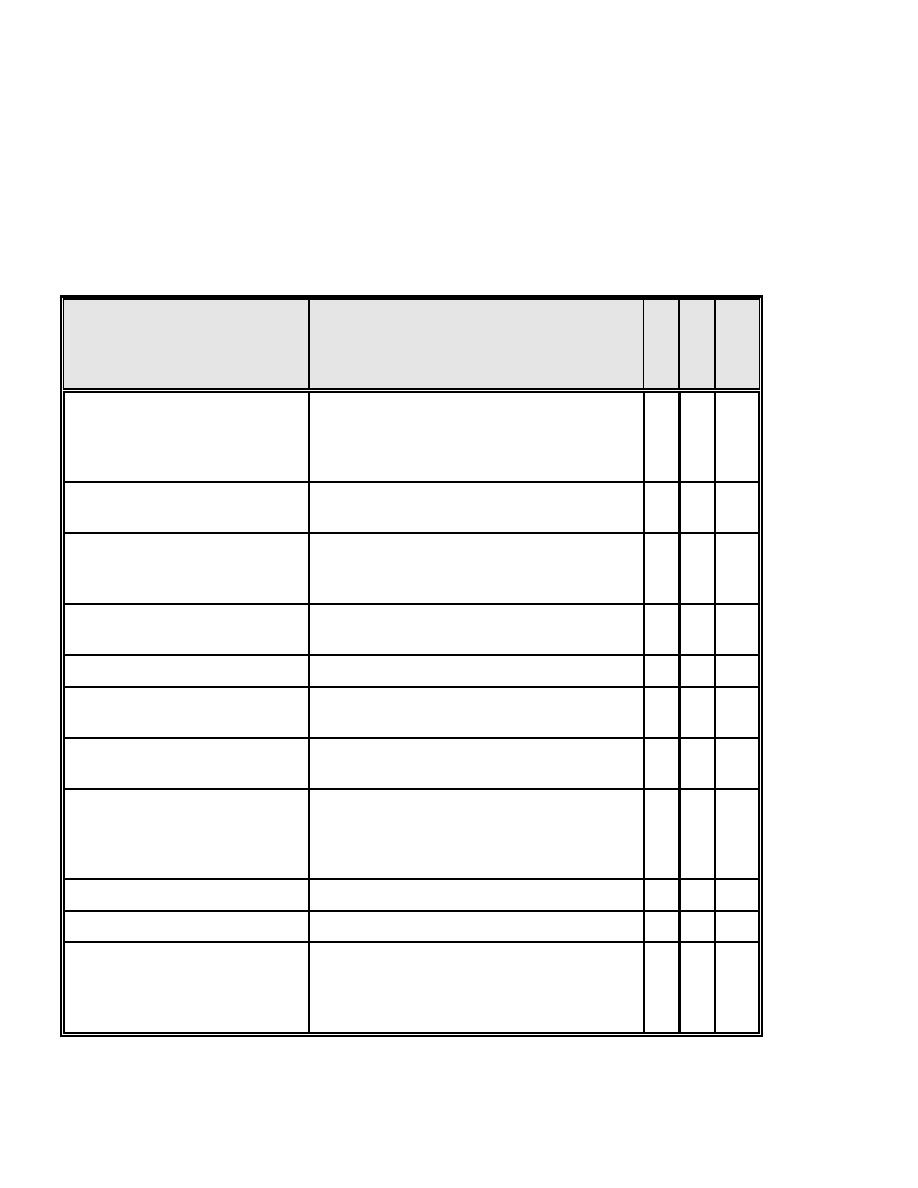Module B - hdbk10030044Click here to make tpub.com your Home Page Page Title: Module B Back | Up | NextWeb www.tpub.comHome

Information Categories
Aerographer
Automotive
Aviation
Construction
Diving
Draftsman
Engineering
Electronics
Food and Cooking
Logistics
Math
Medical
Music
Nuclear Fundamentals
Photography
ReligionDOE-HDBK-1003-96
APPENDIX C
C.1.2 Module B
Instruction should enable the trainee to explain and use the terms, units and definitions and to
successfully apply the concepts on the job.
C.1.3 Mathematics Matrix
SUBJECT
TOPIC
E
M
I
L
E
&
E
C
C
C
H
Basic mathematical concepts
Basic arithmetic functions
B
B
B
Percentage
B
B
B
Square root
B
A
B
Averages
B
B
B
Scientific notation
Conversion of numbers
A
-
B
Application of scientific notation
A
-
B
Dimensional analysis
Unit conversions
B
B
B
Unit modifiers
B
-
B
Metric measurements
B
A
B
Algebra
Basic equation solving
B
A
B
-
-
A
Trigonometry
Basic relationships
A
A
A
Basic relationships
B
A
B
Vectors
B
A
B
Concept of rate of change
-
-
A
Concept of integration
-
-
A
Analysis of graphs and control
Obtaining information from graphs
B
B
B
charts
Rectangular coordinate system
-
-
B
Polar coordinate system
B
A
B
Logarithmic coordinate system
-
-
B
Nomogram
Obtaining information from a nomogram
B
B
B
Exponent base
"E" exponents (Natural/Napierian)
-
-
B
Numbering systems
Binary system
A
-
B
Octal system
-
-
BIntegrated Publishing, Inc. - A (SDVOSB) Service Disabled Veteran Owned Small Business